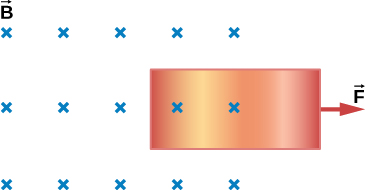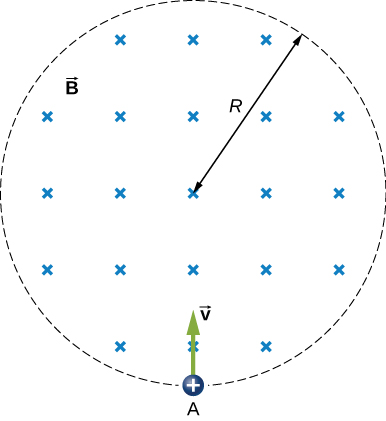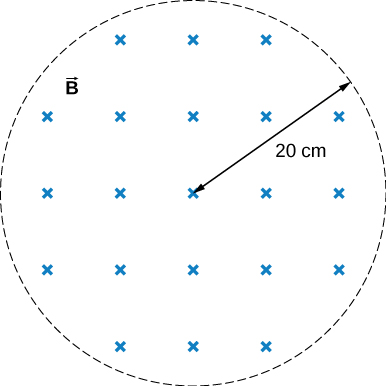# 13.4 Induced electric fields  (Page 3/4)

 Page 3 / 4

## Summary

• A changing magnetic flux induces an electric field.
• Both the changing magnetic flux and the induced electric field are related to the induced emf from Faraday’s law.

## Conceptual questions

Is the work required to accelerate a rod from rest to a speed v in a magnetic field greater than the final kinetic energy of the rod? Why?

The work is greater than the kinetic energy because it takes energy to counteract the induced emf.

The copper sheet shown below is partially in a magnetic field. When it is pulled to the right, a resisting force pulls it to the left. Explain. What happen if the sheet is pushed to the left?## Problems

Calculate the induced electric field in a 50-turn coil with a diameter of 15 cm that is placed in a spatially uniform magnetic field of magnitude 0.50 T so that the face of the coil and the magnetic field are perpendicular. This magnetic field is reduced to zero in 0.10 seconds. Assume that the magnetic field is cylindrically symmetric with respect to the central axis of the coil.

4.67 V/m

The magnetic field through a circular loop of radius 10.0 cm varies with time as shown in the accompanying figure. The field is perpendicular to the loop. Assuming cylindrical symmetry with respect to the central axis of the loop, plot the induced electric field in the loop as a function of time.

The current I through a long solenoid with n turns per meter and radius R is changing with time as given by dI / dt . Calculate the induced electric field as a function of distance r from the central axis of the solenoid.

Inside, $B={\mu }_{0}nI\text{,}\phantom{\rule{0.5em}{0ex}}\oint \stackrel{\to }{E}·d\stackrel{\to }{l}=\left(\pi {r}^{2}\right){\mu }_{0}n\frac{dI}{dt},$ so, $E=\frac{{\mu }_{0}nr}{2}·\frac{dI}{dt}$ (inside). Outside, $E\left(2\pi r\right)=\pi {R}^{2}{\mu }_{0}n\frac{dI}{dt},$ so, $E=\frac{{\mu }_{0}n{R}^{2}}{2r}·\frac{dI}{dt}$ (outside)

Calculate the electric field induced both inside and outside the solenoid of the preceding problem if $I={I}_{0}\phantom{\rule{0.2em}{0ex}}\text{sin}\phantom{\rule{0.2em}{0ex}}\omega t.$

Over a region of radius R , there is a spatially uniform magnetic field $\stackrel{\to }{B}.$ (See below.) At $t=0$ , $B=1.0\phantom{\rule{0.2em}{0ex}}\text{T,}$ after which it decreases at a constant rate to zero in 30 s. (a) What is the electric field in the regions where $r\le R$ and $r\ge R$ during that 30-s interval? (b) Assume that $R=10.0\phantom{\rule{0.2em}{0ex}}\text{cm}$ . How much work is done by the electric field on a proton that is carried once clock wise around a circular path of radius 5.0 cm? (c) How much work is done by the electric field on a proton that is carried once counterclockwise around a circular path of any radius $r\ge R$ ? (d) At the instant when $B=0.50\phantom{\rule{0.2em}{0ex}}\text{T}$ , a proton enters the magnetic field at A , moving a velocity $\stackrel{\to }{v}$ $\left(v=5.0\phantom{\rule{0.2em}{0ex}}×\phantom{\rule{0.2em}{0ex}}{10}^{6}\phantom{\rule{0.2em}{0ex}}\text{m}\text{/}\text{s}\right)$ as shown. What are the electric and magnetic forces on the proton at that instant?a. ${E}_{\text{inside}}=\frac{r}{2}\phantom{\rule{0.2em}{0ex}}\frac{dB}{dt}$ , ${E}_{\text{outside}}=\frac{{r}^{2}}{2R}\phantom{\rule{0.2em}{0ex}}\frac{dB}{dt}$ ; b. $W=4.19\phantom{\rule{0.2em}{0ex}}×\phantom{\rule{0.2em}{0ex}}{10}^{-23}\phantom{\rule{0.2em}{0ex}}\text{J}$ ; c. 0 J; d. ${F}_{\text{mag}}=4\phantom{\rule{0.2em}{0ex}}×\phantom{\rule{0.2em}{0ex}}{10}^{-13}\phantom{\rule{0.2em}{0ex}}\text{N},$ ${F}_{\text{elec}}=2.7\phantom{\rule{0.2em}{0ex}}×\phantom{\rule{0.2em}{0ex}}{10}^{-22}\phantom{\rule{0.2em}{0ex}}\text{N}$

The magnetic field at all points within the cylindrical region whose cross-section is indicated in the accompanying figure starts at 1.0 T and decreases uniformly to zero in 20 s. What is the electric field (both magnitude and direction) as a function of r , the distance from the geometric center of the region?The current in a long solenoid of radius 3 cm is varied with time at a rate of 2 A/s. A circular loop of wire of radius 5 cm and resistance $2\phantom{\rule{0.2em}{0ex}}\text{Ω}$ surrounds the solenoid. Find the electrical current induced in the loop.

$7.1\phantom{\rule{0.2em}{0ex}}\mu \text{A}$

The current in a long solenoid of radius 3 cm and 20 turns/cm is varied with time at a rate of 2 A/s. Find the electric field at a distance of 4 cm from the center of the solenoid.

what are waves
In physics, mathematics, and related fields, a wave is a propagating dynamic disturbance (change from equilibrium) of one or more quantities
Discuss how would orient a planar surface of area A in a uniform electric field of magnitude E0 to obtain (a) the maximum flux and (b) the minimum flux through the area.
I'm just doing the first 3 with this message. but thankyou for the time your obviously intending to support us with. viva la accumulation
Marcel
Find the net capacitance of the combination of series and parallel capacitors shown belo
what is ohm?
calculate ideal gas pressure of 0.300mol,v=2L T=40°c
what is principle of superposition
what are questions that are likely to come out during exam
what is electricity
watt is electricity.
electricity ka full definition with formula
Jyoti
If a point charge is released from rest in a uniform electric field will it follow a field line? Will it do so if the electric field is not uniform?
Maxwell's stress tensor is
Yes
doris
neither vector nor scalar
Anil
if 6.0×10^13 electrons are placed on a metal sphere of charge 9.0micro Coulombs, what is the net charge on the sphere
18.51micro Coulombs
ASHOK
Is it possible to find the magnetic field of a circular loop at the centre by using ampere's law?
Is it possible to find the magnetic field of a circular loop at it's centre?
yes
Brother
The density of a gas of relative molecular mass 28 at a certain temperature is 0.90 K kgmcube.The root mean square speed of the gas molecules at that temperature is 602ms.Assuming that the rate of diffusion of a gas in inversely proportional to the square root of its density,calculate the density of
A hot liquid at 80degree Celsius is added to 600g of the same liquid originally at 10 degree Celsius. when the mixture reaches 30 degree Celsius, what will be the total mass of the liquid?
Under which topic
doris
what is electrostatics
Study of charges which are at rest
himanshu

#### Get Jobilize Job Search Mobile App in your pocket Now!By OpenStaxBy Mucho MizindukoBy OpenStaxBy OpenStaxByBy OpenStaxBy Rylee MinllicBy Sandy YamaneBy Madison ChristianBy OpenStax